SYLLABUS  Previous: 2 FINITE DIFFERENCES  Up: 2 FINITE DIFFERENCES  Next: 2.2 Explicit 3 levels

## 2.1 Explicit 2 levels

Slide : [ 2 levels Scheme - Code - Run - Stability - Upwind || VIDEO login]

A spatial difference to the left for the advection (1.3.1#eq.1) and a centered difference for the diffusion (1.3.2#eq.1) for example yields an explicit scheme for the advection-diffusion equation that involves only two time levels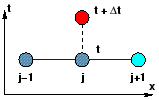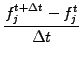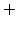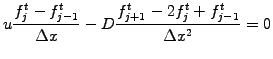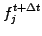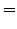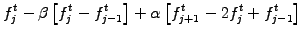(2.1.0#eq.1)

The Courant-Friedrichs-Lewy (CFL) number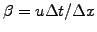and the coefficient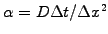measure typical advection and diffusion velocities relative to the characteristic propagation speed of the mesh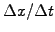. For every step in time, a new value of the solution fp[j] is obtained explicitly from a linear combination involving the nearest neighbors f[j-1],f[j],f[j+1], which are known either from a previous step or the initial condition. This scheme has been implemented in JBONE as
      for (int j=1; j<n; j++) {
fp[j]=f[j] -beta *(f[j]-f[j-1])+alpha*(f[j+1]-2.*f[j]+f[j-1]); }
fp=f   -beta *(f-f[ n ])+alpha*(f[ 1 ]-2.*f+f[ n ]);
fp[n]=f[n]   -beta *(f[n]-f[n-1])+alpha*(f[ 0 ]-2.*f[n]+f[n-1]);

where the last two statements take care of the periodicity.

Sharp variations of the solution should be AVOIDED to produce physically meaningful results; to study the numerical properties of a approximation, it is however often illuminating to initialize a box function and check how the intrinsic numerical dispersion / damping of a scheme affects the superposition of both the short and the long wavelengths. The example below shows the result of an evolution with a constant advection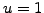and no physical diffusion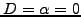.

After 128 steps of duration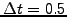, the pulse propagates exactly once accross the periodic domain, which is here discretized with 64 mesh points homogeneously distributed over the length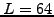so that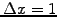. The lowest order moment (density, (1.2.5#eq.1)) is conserved to a very good accuracy (as can be judged from the JBONE monitor under Mom[%]) and the function remains positive everywhere as it should(as can be judged from Min) . The shape, however, is strongly affected as the sharp edges are smoothed out due intrinsic numerical damping.

 Numerical experiments: explicit 2-levels Change the initial condition from Box to Cosine, and vary the wavelength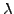(parameter ICWavelength) from 2-64 mesh points per wavelength to verify that it is the short wavelengths associated with steep edges that are heavily damped: exactly what you expect from diffusion (1.3.2#eq.4) except that with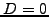, this is here a numerical artifact. Without special care, numerical damping can easily cover the physical process you want to model! Looking for a quick fix, you reduce the time step and the CFL number from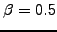down 0.1. What happens ? Adding to the confusion, increase the time step to exactly 1 and check what happens.

The properties of a FD approximation are best understood from a numerical dispersion / Von Neuman stability analysis, which is carried out on a homogeneous grid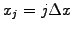using a hamonic ansatz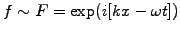. Define the amplification factor as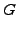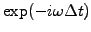(2.1.0#eq.2)

and simplify by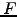to cast the dispersion relation into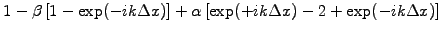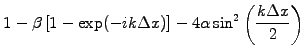(2.1.0#eq.3)

Figure 2.1#fig.2 illustrates with vectors in the complex plane how the first three term are combined in the presence of advection only (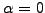).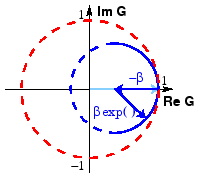Short wavelengths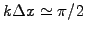are heavily damped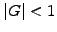(area inside the outer circle) if the CFL number is smaller than unity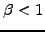; for values exceeding the magic time step where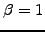, the short wavelengths however grow into a so-called numerical instability. Reload the initial applet parameters and try to reproduce this in JBONE.

 Numerical experiments: explicit 2-levels (cont) Increase the TimeStep=1.01 and observe the slowly growing mode with 2 mesh points per wavelength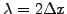, i.e.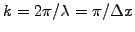. Starting from the initial applet parameters, take two steps using a Cosine with ICWavelength=8 mesh points per wavelength to determine the value of the physical Diffusion that approximatively doubles the damping observed when- e.g. doubling the value of the Min value displayed in the applet. Choose the value of the numerical parameters (MeshPoints, TimeStep) to compute a physical evolution of a Gaussian pulse with a ICWidth=2.5 when the advection Velocity=1 and the Diffusion=0.1 is relatively small. Make sure that the numerical dispersion and damping are both small in comparison with the physical effect you try to reproduce.

For a diffusive process (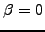), the dispersion relation (2.1#eq.3) shows that the 2 levels scheme is stable for all wavelengths provided that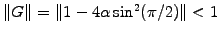, i.e.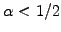. Although the superposition of advection and diffusion is slightly more limiting, the overall conditions for numerical stability can conveniently be summarized as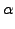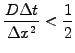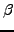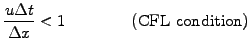(2.1.0#eq.4)

It is finally worth pointing out that a backward finite difference for the advection is unstable for negative velocities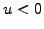; this defect of non-centered schemes is sometimes cured with an upwind difference, which takes the finite difference forward or backward depending on the local direction of propagation (exercise 2.01).

SYLLABUS  Previous: 2 FINITE DIFFERENCES  Up: 2 FINITE DIFFERENCES  Next: 2.2 Explicit 3 levels